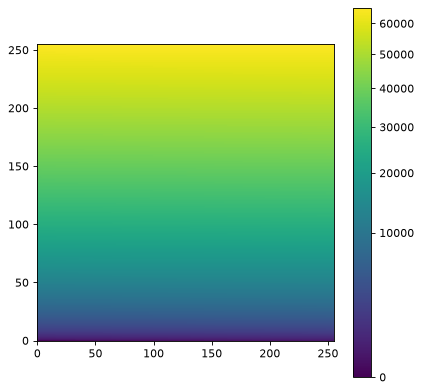# imshow_norm¶

astropy.visualization.imshow_norm(data, ax=None, imshow_only_kwargs={}, **kwargs)[source]

A convenience function to call matplotlib’s matplotlib.pyplot.imshow function, using an ImageNormalize object as the normalization.

Parameters
data2D or 3D array_like - see imshow

The data to show. Can be whatever imshow and ImageNormalize both accept.

axNone or Axes, optional

If None, use pyplot’s imshow. Otherwise, calls imshow method of the supplied axes.

imshow_only_kwargsdict, optional

Deprecated since Astropy v4.1. Note that settting both norm and vmin/vmax is deprecated in matplotlib >= 3.3.

Arguments to be passed directly to imshow without first trying ImageNormalize. This is only for keywords that have the same name in both ImageNormalize and imshow - if you want to set the imshow keywords only, supply them in this dictionary.

kwargsdict, optional

All other keyword arguments are parsed first by the ImageNormalize initializer, then to imshow.

Returns
resulttuple

A tuple containing the AxesImage generated by imshow as well as the ImageNormalize instance.

Notes

The norm matplotlib keyword is not supported.

Examples

import numpy as np
import matplotlib.pyplot as plt
from astropy.visualization import (imshow_norm, MinMaxInterval,
SqrtStretch)

# Generate and display a test image
image = np.arange(65536).reshape((256, 256))
fig = plt.figure()